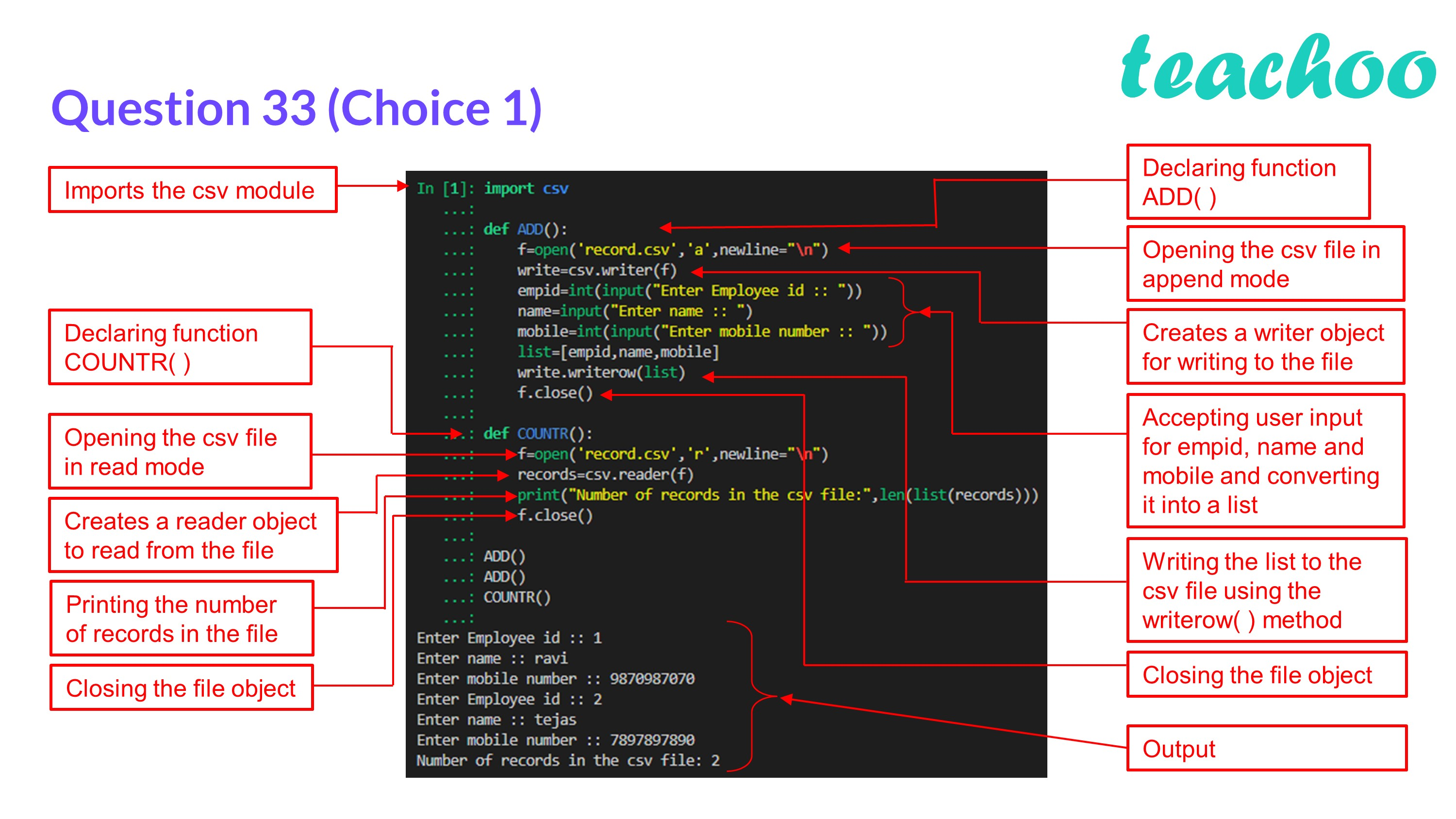CBSE Class 12 Sample Paper for 2023 Boards

Computer Science - Class 12
Solutions to CBSE Sample Paper - Computer Science Class 12

## (ii) COUNTR() – To count the number of records present in the CSV file named ‘record.csv’.

Advantages of using a csv file for permanent storage are:

• It is human readable and easy to edit.
• It is compact.
• It is faster to handle.

Code:

import csv

def ``` ADD ``` ():

f=open( ``` 'record.csv','a', ``` newline= ``` "\n" ``` )

write=csv.writer(f)

empid=int(input( ``` "Enter Employee id :: " ``` ))

name=input( ``` "Enter name :: " ``` )

mobile=int(input( ``` "Enter mobile number :: " ``` ))

list=[empid,name,mobile]

write.writerow(list)

f.close()

def ``` COUNTR ``` ():

f=open( ``` 'record.csv','r' ``` ,newline= ``` "\n" ``` )

print( ``` "Number of records in the csv file:" ``` ,len(list(records)))

f.close()

COUNTR()Learn in your speed, with individual attention - Teachoo Maths 1-on-1 Class